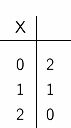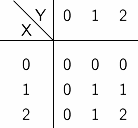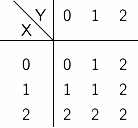Time Limit : sec, Memory Limit : KB

# Problem C: How can I satisfy thee? Let me count the ways...

Three-valued logic is a logic system that has, in addition to "true" and "false", "unknown" as a valid value. In the following, logical values "false", "unknown" and "true" are represented by 0, 1 and 2 respectively.

Let "-" be a unary operator (i.e. a symbol representing one argument function) and let both "*" and "+" be binary operators (i.e. symbols representing two argument functions). These operators represent negation (NOT), conjunction (AND) and disjunction (OR) respectively. These operators in three-valued logic can be defined in Table C-1.

Table C-1: Truth tables of three-valued logic operators
 -X (X*Y) (X+Y)Let P, Q and R be variables ranging over three-valued logic values. For a given formula, you are asked to answer the number of triples (P,Q,R) that satisfy the formula, that is, those which make the value of the given formula 2. A formula is one of the following form (X and Y represent formulas).

• Constants: 0, 1 or 2
• Variables: P, Q or R
• Negations: -X
• Conjunctions: (X*Y)
• Disjunctions: (X+Y)

Note that conjunctions and disjunctions of two formulas are always parenthesized.

For example, when formula (P*Q) is given as an input, the value of this formula is 2 when and only when (P,Q,R) is (2,2,0), (2,2,1) or (2,2,2). Therefore, you should output 3.

### Input

The input consists of one or more lines. Each line contains a formula. A formula is a string which consists of 0, 1, 2, P, Q, R, -, *, +, (, ). Other characters such as spaces are not contained. The grammar of formulas is given by the following BNF.

<formula> ::= 0 | 1 | 2 | P | Q | R |
-<formula> | (<formula>*<formula>) | (<formula>+<formula>)


All the formulas obey this syntax and thus you do not have to care about grammatical errors. Input lines never exceed 80 characters.

Finally, a line which contains only a "." (period) comes, indicating the end of the input.

### Output

You should answer the number (in decimal) of triples (P,Q,R) that make the value of the given formula 2. One line containing the number should be output for each of the formulas, and no other characters should be output.

### Sample Input

(P*Q)
(--R+(P*Q))
(P*-P)
2
1
(-1+(((---P+Q)*(--Q+---R))*(-R+-P)))
.


### Output for the Sample Input

3
11
0
27
0
7